Ex 10.2

Chapter 10 Class 7 Algebraic Expressions
Serial order wise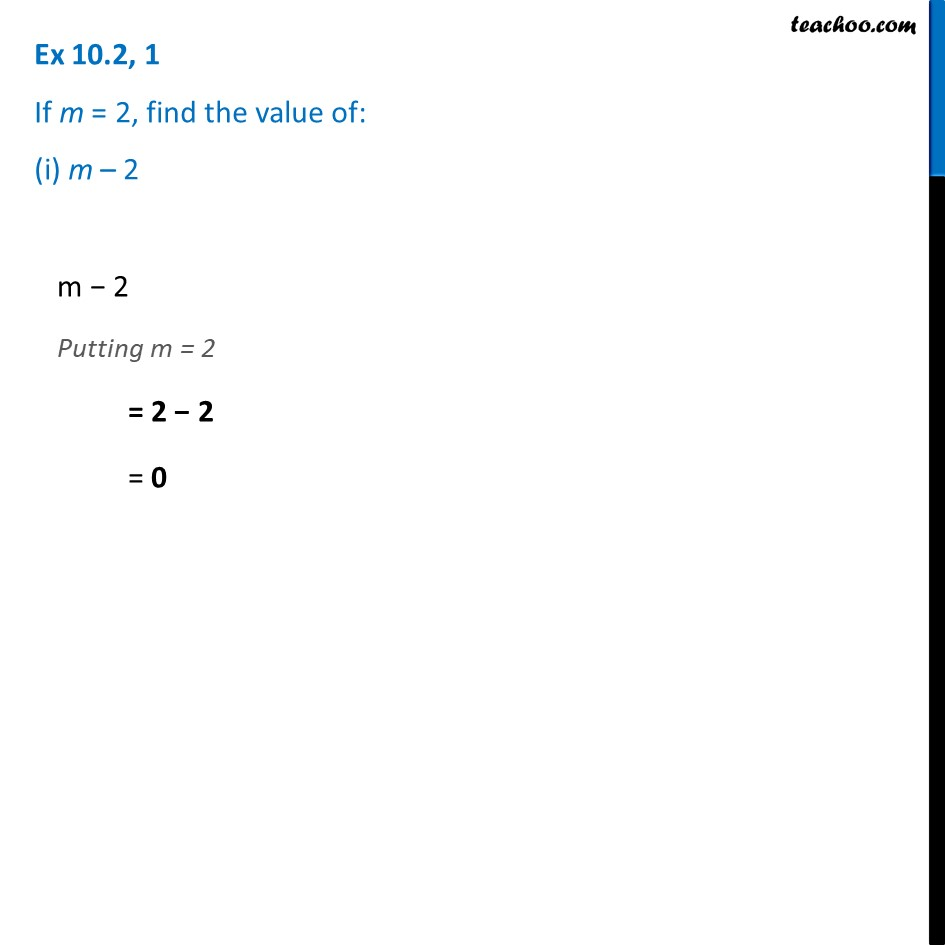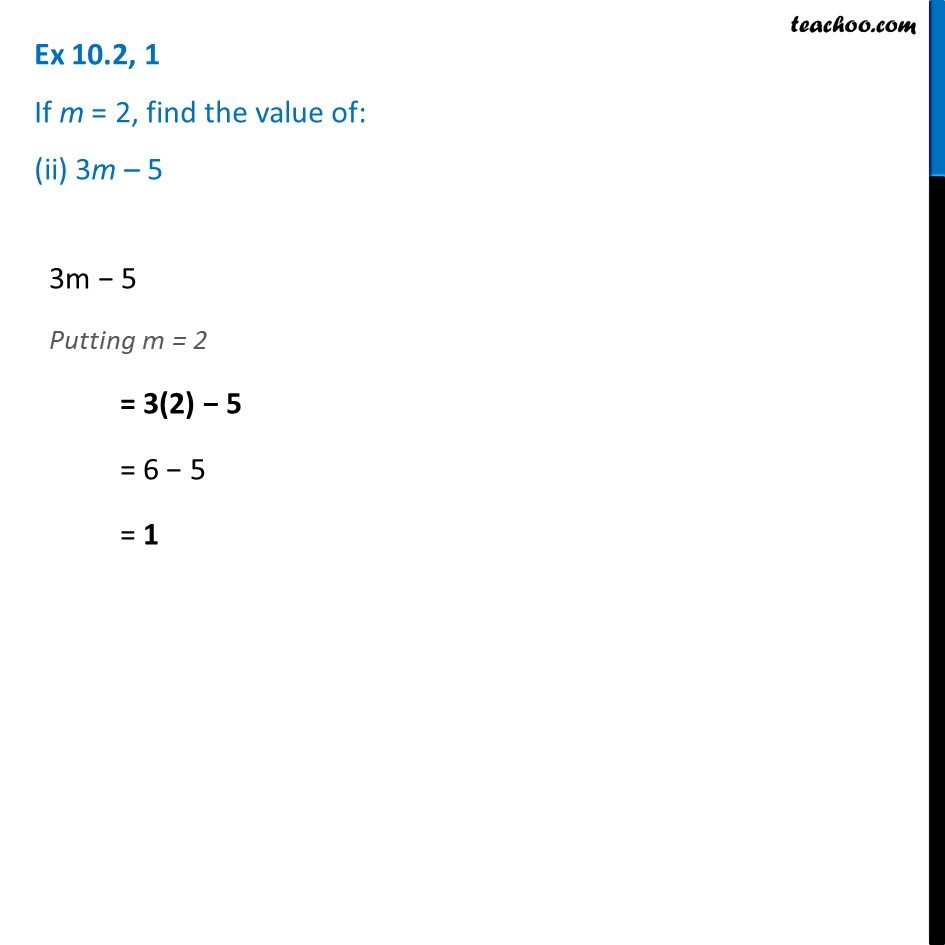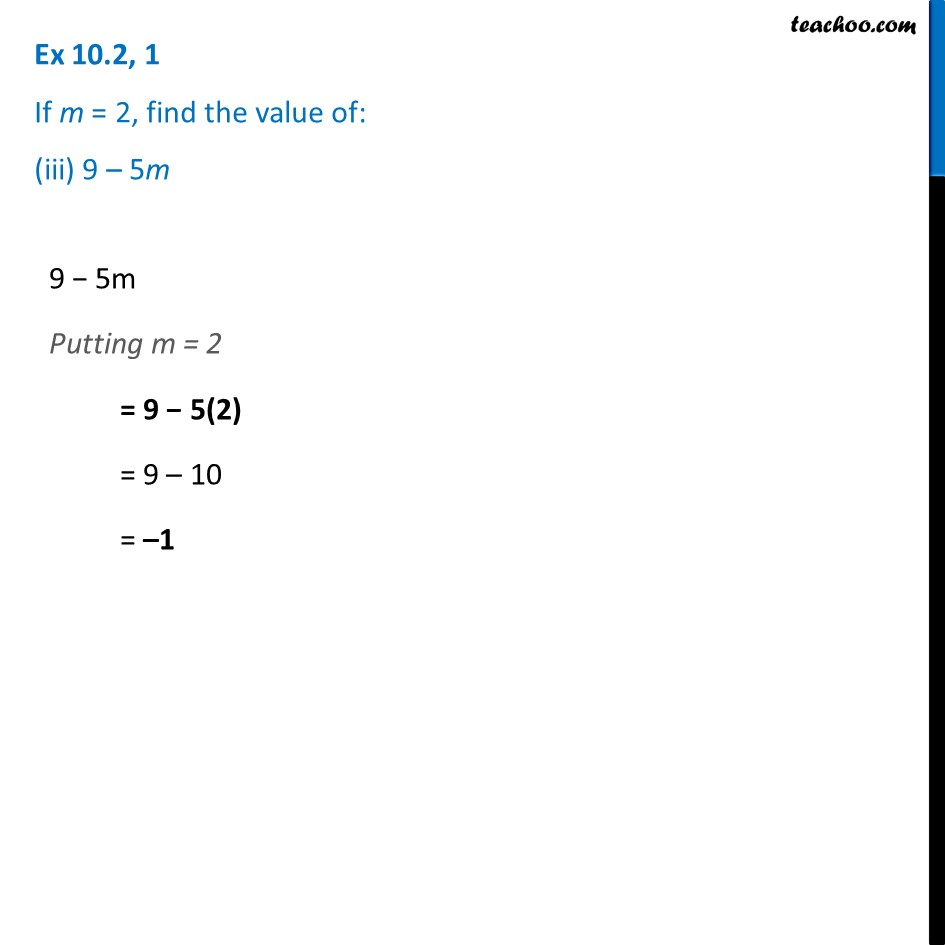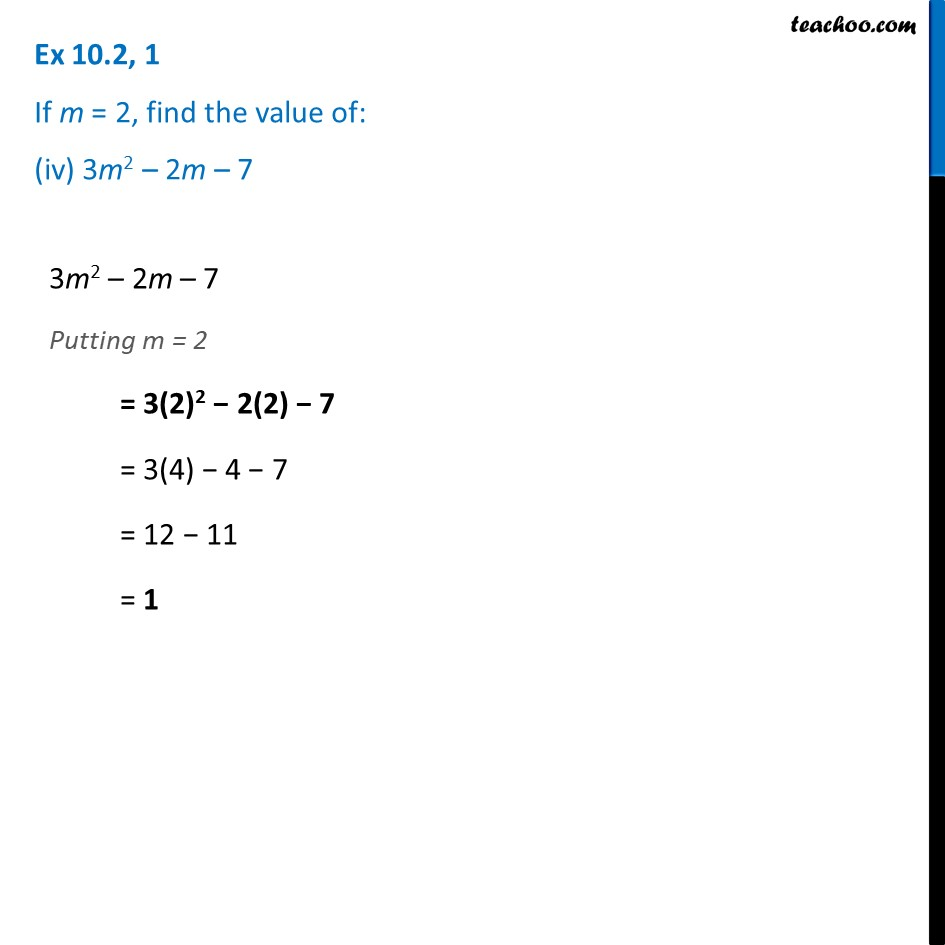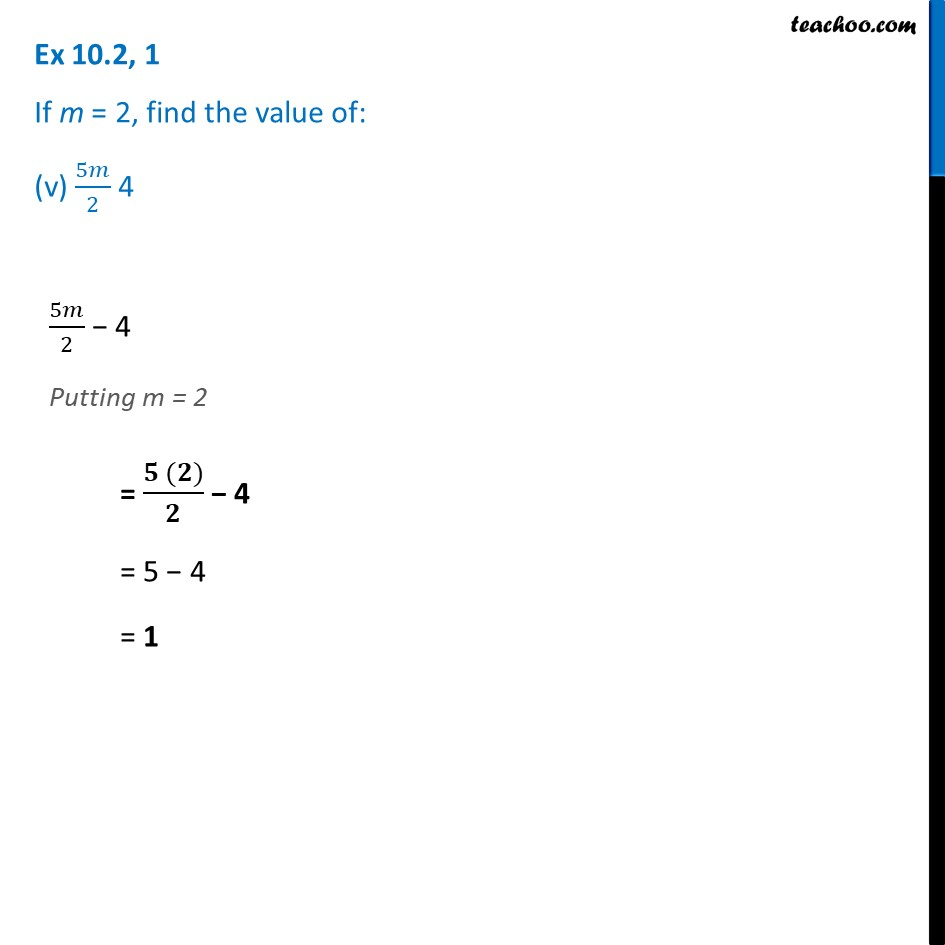Learn in your speed, with individual attention - Teachoo Maths 1-on-1 Class

### Transcript

Ex 10.2, 1 If m = 2, find the value of: (i) m – 2 m − 2 Putting m = 2 = 2 − 2 = 0 Ex 10.2, 1 If m = 2, find the value of: (ii) 3m – 5 3m − 5 Putting m = 2 = 3(2) − 5 = 6 − 5 = 1 Ex 10.2, 1 If m = 2, find the value of: (iii) 9 – 5m9 − 5m Putting m = 2 = 9 − 5(2) = 9 – 10 = –1 Ex 10.2, 1 If m = 2, find the value of: (iv) 3m2 – 2m – 7 3m2 – 2m – 7 Putting m = 2 = 3(2)2 − 2(2) − 7 = 3(4) − 4 − 7 = 12 − 11 = 1 Ex 10.2, 1 If m = 2, find the value of: (v) 5𝑚/2 4 5𝑚/2 − 4 Putting m = 2 = (𝟓 (𝟐))/𝟐 − 4 = 5 − 4 = 1 Ex 10.2, 1 If m = 2, find the value of: (v) 5𝑚/2 4 5𝑚/2 − 4 Putting m = 2 = (𝟓 (𝟐))/𝟐 − 4 = 5 − 4 = 1